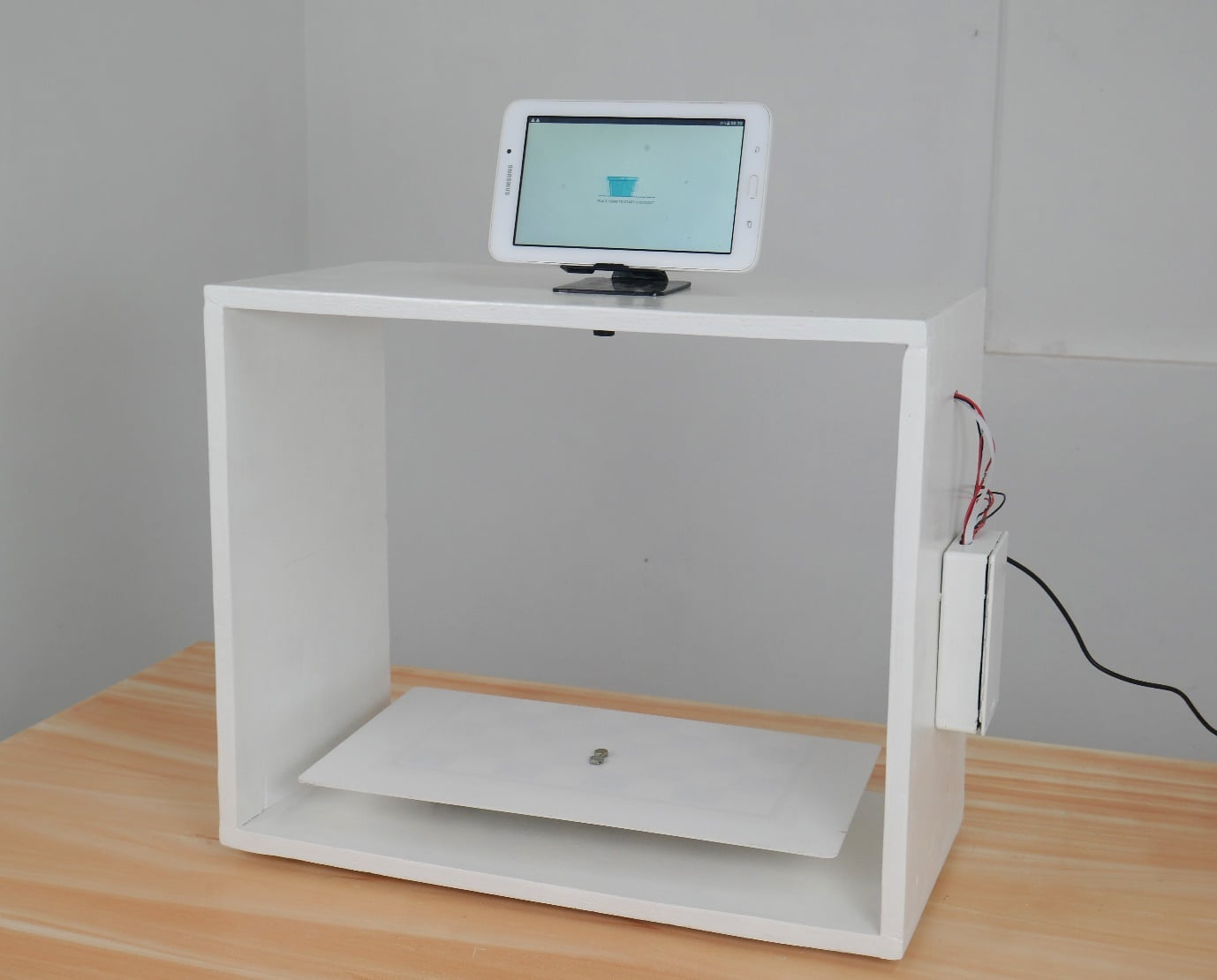1631 1
Nekhil Ravi

## Auto Bill

AutoBill is an AI-powered autonomous checkout system for retail stores, that combines the power of...

##### Description:-

AutoBill is an AI-powered autonomous checkout system for retail stores, that combines the power of computer vision and machine learning to provide an amazing shopping experience. AutoBill provides a faster checkout shopping experience to minimize human interactions in the store to keep shoppers and employees safer during the pandemic.

AutoBill uses computer vision and machine learning to visually detect and instantly identify the items placed and the weight sensor measure the weights of the things placed on the counter-top. Once the items are identified, things are automatically added to the cart and the bill is generated instantaneously. QR code for payment is generated and users can pay the bill by scanning the QR code.

Features:

• AI powered
• Instant checkout
• Contact-free checkout
• Easy deployment

So Let's dive in…

HARDWARE :

• Raspberry Pi 3B
• REES52 5 Megapixel 160° degrees
• HX711 Breakout board
• WS2812B RGB LED Strip
• 5V 2A power supply

SOFTWARE :

• Edge Impulse
• Raspbian OS
• VS code editor

Work Flow :

Let's have a look at the logical flow of Auto Bill.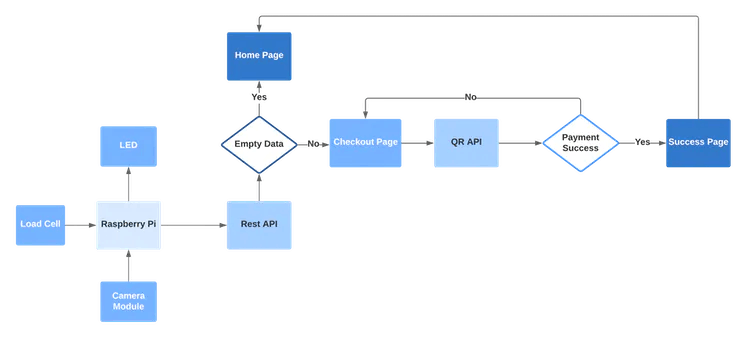Object detection :

Edge Impulse is one of the leading development platforms for machine learning on edge devices, free for developers and trusted by enterprises. Here we are using machine learning to build a system that can recognize the products available in the shops. Then we deploy the system on the Raspberry Pi 3B. To make the machine learning model it's important to have a lot of images of the products. When training the model, these product images are used to let the model distinguish between them. Make sure you have a wide variety of angles and zoom levels of the products which are available in the shops. For the data acquisition, you can capture data from any device or development board, or admin/upload your existing datasets. So here we captured data from our device and labelled them into the three categories.

So here we have four different labels Apple,Coke and Lays. So the system will only detect these items. If you want to recognize any other objects other than these you need to admin/upload or capture that particular item.

With the training set in place, you can design an impulse. An impulse takes the raw data, adjusts the image size, uses a preprocessing block to manipulate the image, and then uses a learning block to classify new data. Preprocessing blocks always return the same values for the same input (e.g. convert a color image into a grayscale one), while learning blocks learn from past experiences.

For this system, we'll use the 'Images' preprocessing block. This block takes in the color image, optionally makes the image grayscale, and then turns the data into a features array. Then we'll use a 'Transfer Learning' learning block, which takes all the images in and learns to distinguish between the two ('coffee', 'lamp') classes.

In the studio go to Create impulse, set the image width and image height to 96, the 'resize mode' to Fit shortest axis, and add the 'Images' and 'Object Detection (Images)' blocks. Then click Save impulse.

Then in the image tab, you can see the raw and processed features of every image. You can use the options to switch between 'RGB' and 'Grayscale' mode, but for now, leave the color depth on 'RGB' and click Save parameters.

This will send you to the Feature generation screen.

Click Generate features to start the process.

Afterward the 'Feature explorer' will load. This is a plot of all the data in your dataset. Because images have a lot of dimensions (here: 96x96x3=27648 features) we run a process called 'dimensionality reduction' on the dataset before visualizing this. Here the 27648 features are compressed down to just 3, and then clustered based on similarity. Even though we have little data you can already see the clusters forming and can click on the dots to see which image belongs to which dot.

With all data processed it's time to start training a neural network. Neural networks are a set of algorithms, modeled loosely after the human brain, that are designed to recognize patterns. The network that we're training here will take the image data as an input, and try to map this to one of the three classes.

It's very hard to build a good working computer vision model from scratch, as you need a wide variety of input data to make the model generalize well, and training such models can take days on a GPU. To make this easier and faster we are using transfer learning. This lets you piggyback on a well-trained model, only retraining the upper layers of a neural network, leading to much more reliable models that train in a fraction of the time and work with substantially smaller datasets.

To configure the transfer learning model, click Object detection in the menu on the left. Here you can select the base model (the one selected by default will work, but you can change this based on your size requirements), and set the rate at which the network learns.

Leave all settings as-is, and click Start training. After the model is done you'll see accuracy numbers below the training output. We have now trained our model.

With the model trained let's try it out on some test data. When collecting the data we split the data up between training and a testing dataset. The model was trained only on the training data, and thus we can use the data in the testing dataset to validate how well the model will work in the real world. This will help us ensure the model has not learned to overfit the training data, which is a common occurrence.

To validate your model, go to Model testing and select Classify all. Here we hit 93.75% precision, which is great for a model with so little data.

To see classification in detail, click the three dots next to an item, and select Show classification. This brings you to the Live classification screen with much more details on the file (you can also capture new data directly from your development board from here). This screen can help you determine why items were misclassified.

With the impulse designed, trained, and verified you can deploy this model back to your device. This makes the model run without an internet connection, minimizes latency, and runs with minimum power consumption. Edge Impulse can package up the complete impulse - including the preprocessing steps, neural network weights, and classification code - in a single C++ library or model file that you can include in your embedded software.

Reasons for choosing hardware and software & Setup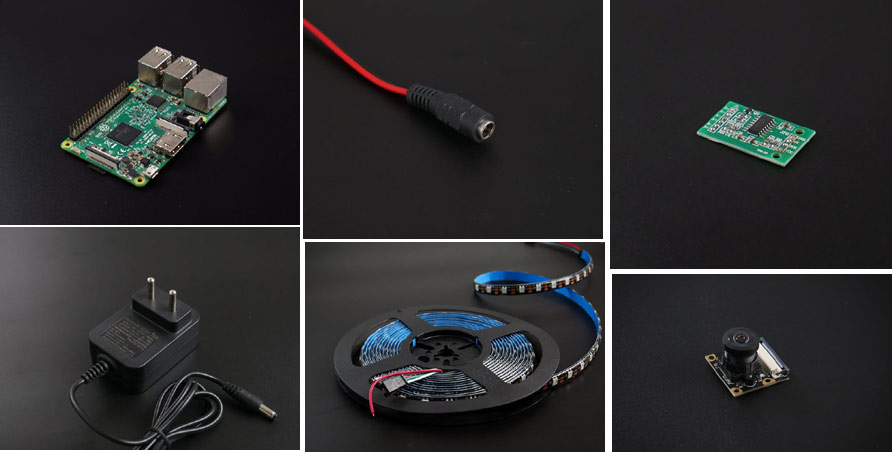### RASPBERRY PI 3B

The Raspberry Pi 3B is the powerful development of the extremely successful credit card-sized computer system. The brain of the device is Raspberry Pi. All major processes are carried out by this device.

If you don't know how to set up the Pi just go here.

To set this device up in Edge Impulse, run the following commands:

```curl` -sL https://deb.nodesource.com/setup_`12`.x | sudo bash -`

`sudo apt install -y gcc g++ make build-essential nodejs sox gstreamer`1`.`0`-tools gstreamer`1`.`0`-plugins-good gstreamer`1`.`0`-plugins-base gstreamer`1`.`0`-plugins-base-apps`

`npm config set user root && sudo npm install edge-impulse-linux -g --unsafe-perm````

If you have a Raspberry Pi Camera Module, you also need to activate it first. Run the following command:

``sudo raspi-`config`

Use the cursor keys to select and open Interfacing Options, and then select Camera and follow the prompt to enable the camera. Then reboot the Raspberry.

With all software set up, connect your camera to Raspberry Pi and run.

`edge-impulse-linux`

This will start a wizard which will ask you to log in and choose an Edge Impulse project. If you want to switch projects run the command with --clean.

That's all! Your device is now connected to Edge Impulse. To verify this, go to your Edge Impulse project, and click Devices. The device will be listed here.

To run your impulse locally, just connect to your Raspberry Pi again, and run.

`edge-impulse-linux-runner`

Here we are using the Linux Python SDK for integrating the model with the system. For working with the Python SDK you need to have a recent version of the Python(>=3.7)

For installing the SDK for the Raspberry pi, you need to run the following commands.

```sudo` apt-get install libatlas-base-dev libportaudio`0` libportaudio`2` libportaudiocpp`0` portaudio`19`-dev`

`pip`3` install edge_impulse_linux -i https://pypi.python.org/simple````

To classify data,you'll need a model file.We have already trained our model. This model file contains all signal processing code, classical ML algorithms, and neural networks - and typically contains hardware optimizations to run as fast as possible. To download the model file run the below command

``edge-impulse-linux-runner `--download modelfile.eim`

### Camera Module

Here I am using the REES52 5 Megapixel 160° degrees Wide Angle Fish-Eye Camera for the object detection. Due to its high viewing angle, it can cover more area than the normal camera module. The main feature of this camera module is

• Omnivision 5647 sensor in a fixed-focus module.
• The module attaches to Raspberry Pi, by way of a 15 Pin Ribbon Cable, to the dedicated 15-pin MIPI Camera Serial Interface (CSI).
• The CSI bus is capable of extremely high data rates, and it exclusively carries pixel data to the BCM2835 processor.
• The sensor itself has a native resolution of 5 megapixels and has a fixed focus lens onboard.
• The camera supports 1080 p @ 30 fps, 720 p @ 60 fps, and 640 x480 p 60/90 video recording also it is supported in the latest version of Raspbian, the Raspberry Pi's preferred operating system.

For connecting the camera module to the Raspberry pi, we have used 18'' flex cable. There is considerable distance between the Raspberry Pi and the camera module.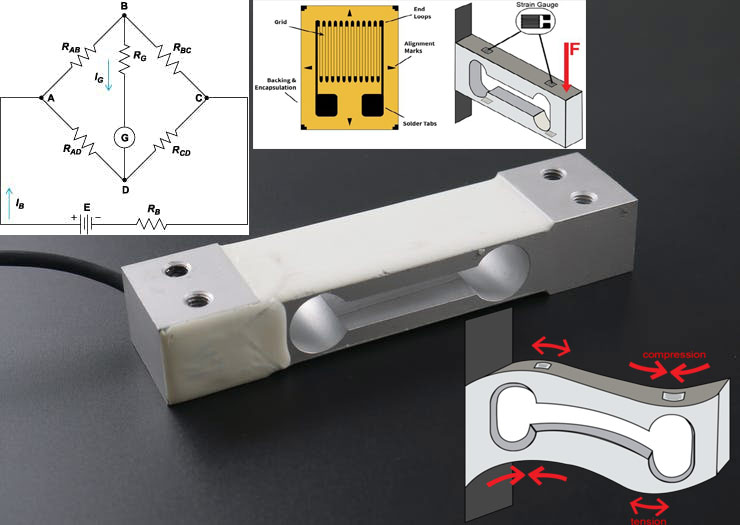Here we use the Load cell to measure the weight of the objects. The load cell is a sensor or a transducer that converts a load or force acting on it into an electronic signal. This electronic signal can be a voltage change, current change, or frequency change depending on the type of load cell and circuitry used. There are many different kinds of load cells.

Here we are using a resistive load cell. Resistive load cells work on the principle of piezo-resistivity. When a load/force/stress is applied to the sensor, it changes its resistance. This change in resistance leads to a change in output voltage when an input voltage is applied. The resistive load cell is made by using an elastic member (with a very highly repeatable deflection pattern) to which a number of strain gauges are attached.

Here we are using a load cell which is having four strain gauges that are bonded to the upper and lower surfaces of the load cell.

When the load is applied to the body of a resistive load cell, as shown above, the elastic member, deflects as shown and creates a strain at those locations due to the stress applied. As a result, two of the strain gauges are in compression, whereas the other two are in tension.

During a measurement, the weight acts on the load cell’s metal spring element and causes elastic deformation. This strain (positive or negative) is converted into an electrical signal by a strain gauge (SG) installed on the spring element. The simplest type of load cell is a bending beam with a strain gauge. We use the Wheatstone bridge circuit to convert this change in strain/resistance into a voltage that is proportional to the load.

The four strain gauges are configured in a Wheatstone Bridge configuration with four separate resistors connected as shown in what is called a Wheatstone Bridge Network. An excitation voltage – usually 5V is applied to one set of corners and the voltage difference is measured between the other two corners. At equilibrium with no applied load, the voltage output is zero or very close to zero when the four resistors are closely matched in value. That is why it is referred to as a balanced bridge circuit.

When the metallic member to which the strain gauges are attached, is stressed by the application of a force, the resulting strain – leads to a change in resistance in one (or more) of the resistors. This change in resistance results in a change in output voltage. This small change in output voltage (usually about 20 mv of the total change in response to full load) can be measured and digitized after careful amplification of the small milli-volt level signals to a higher amplitude 0-5V signal.

HX711 Break outboard

The HX711 module is a Load Cell Amplifier breakout board that allows you to easily read load cells to measure weight. This module uses 24 high-precision A/D converter chips HX711. It is specially designed for the high precision electronic scale design, with two analog input channels, the internal integration of 128 times the programmable gain amplifier. The input circuit can be configured to provide a bridge type pressure bridge (such as pressure, weighing sensor mode), is of high precision, low cost is an ideal sampling front-end module.

HX711 is an IC that allows you to easily integrate load cells into your project. No need for any amplifiers or dual power supply just use this board and you can easily interface it to any micro-controller to measure weight.

The HX711 uses a two-wire interface (Clock and Data) for communication. Compared with other chips, HX711 has added advantages such as high integration, fast response, immunity, and other features improving the total performance and reliability. Finally, it's one of the best choices for electronic enthusiasts. The chip lowers the cost of the electronic scale,at the same time,improving performance and reliability. Its specifications are

• Differential input voltage: ±40mV (Full-scale differential input voltage is ± 40mV)
• Data accuracy: 24 bit (24 bit A / D converter chip.)
• Refresh frequency: 10/80 Hz
• Operating Voltage: 2.7V to 5VDC
• Operating current: <10 mA
• Size: 24x16mm

Calibration

Connect the load cell, HX711 module to the Raspberry pi as per the schematics and run the calibration.py code in raspberry pi. Then you will get the ratio of your own load cell and just put in it your main code. Each load cell ratio would be different on different occasions. Below is my calibration of the load cell.

So weighing with my load cell is 90 % accurate. For connecting the load cell with the raspberry pi using the HX711, I have used the pieces of code by Marcel Zak. You can find his repository here .

### WS2812B RGB LED Strip

The WS2812B 5V Addressable RGB Waterproof LED Strip is extremely flexible, easy to use and each LED of the strip can be controlled separately by using a microcontroller. Each LED has been equipped with an integrated driver that allows you to control the color and brightness of each LED independently. To light up the commodities in the system we have used this RGB led Strip. The combined LED/driver IC on these strips is the extremely compact WS2812B (essentially an improved WS2811 LED driver integrated directly into a 5050 RGB LED), which enables higher LED densities. WS2812B uses a specialized one-wire control interface and requires strict timing.

### Power supply

Here we used a 5V 2A power supply for powering the entire project. We have also used a DC power jack for plugging this adapter.

### Cabinetry

For making the cabinetry for the system, I have used 4 pieces of plywood with the following dimension.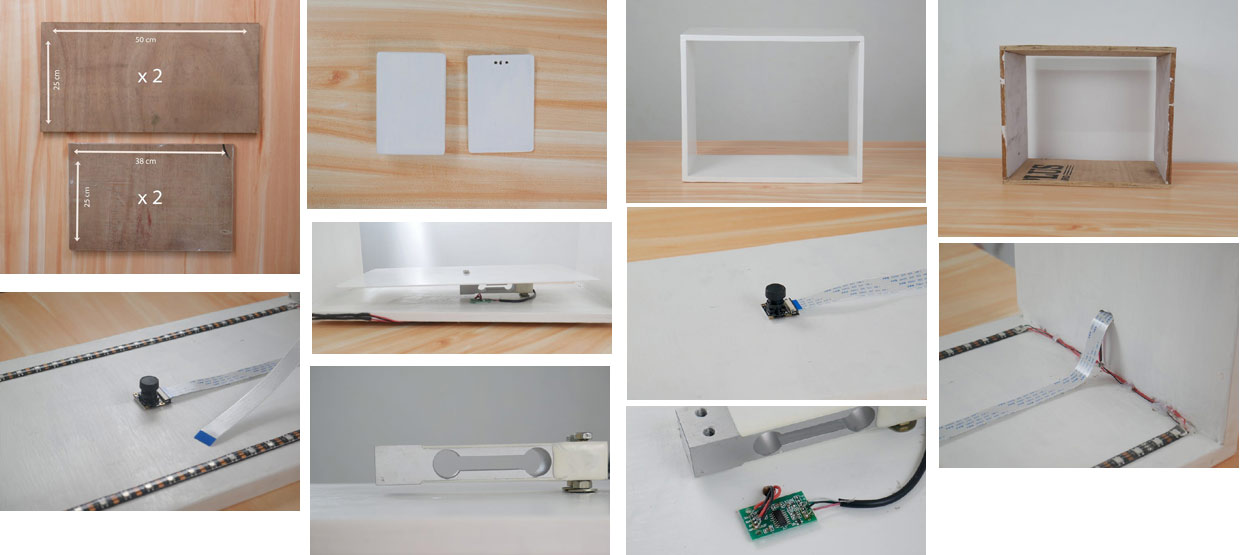These plywoods were sanded with 100 grit sandpaper to get a smooth finish. For placing the load cell on the plywood, I made necessary holes in the wood with a drill after properly measuring the spaces for the component. And these plywoods were made to cabinet properly with pieces of the nail.

After priming the cabinet, I have used wood fillers to fill the voids present in the cabinet. Finally applied Satin finish paint for the cabinet.

Also attached four bushes for the system.

### Securing components

Initially, we attached the load cell on the cabinet with the help of Nuts and bolts.

One end of the load cell should be rigidly connected and another end should be floated in the air, then only we get the proper weight of the object. Then we wired the load cell to the HX711 break-out module by installing it.

Then we pulled out the wire from the HX711 module through a hole nearby it. Then we installed the camera module at the opposite face of the load cell.

Then we attached the led strip parallel to the camera module.

Then these strips were parallelly connected. The flex cable from the camera module and the wire from the strips were pulled out.

For placing the raspberry pi we have used a white painted black box. It is then screwed to the cabinet.

We have also made necessary holes on the white box for connecting components with the raspberry pi.

First, we attached the DC power jack into the box.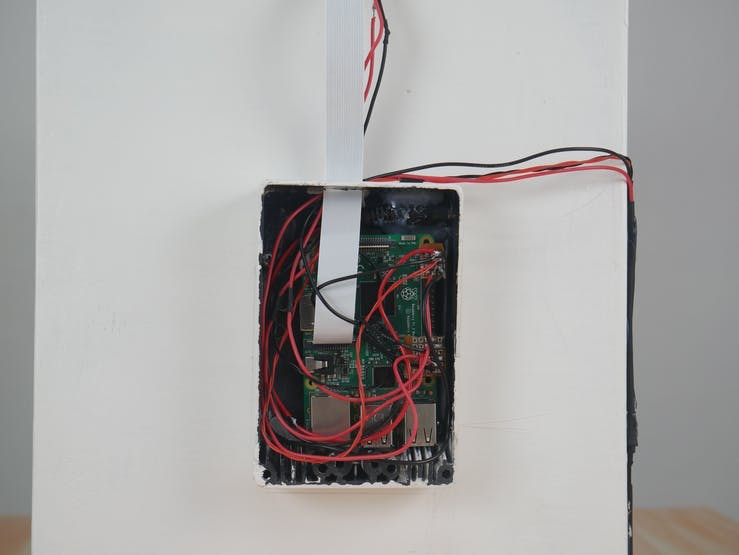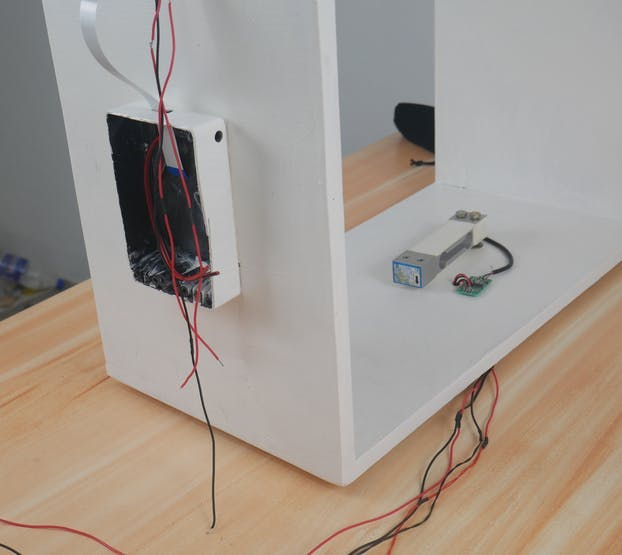Then we attached the raspberry pi and wired the whole components as per the schematics.

Then we attached the lid. Then the base is fixed. Here we used the base piece as the acrylic sheet cut in proper measurement.

The final will look like this.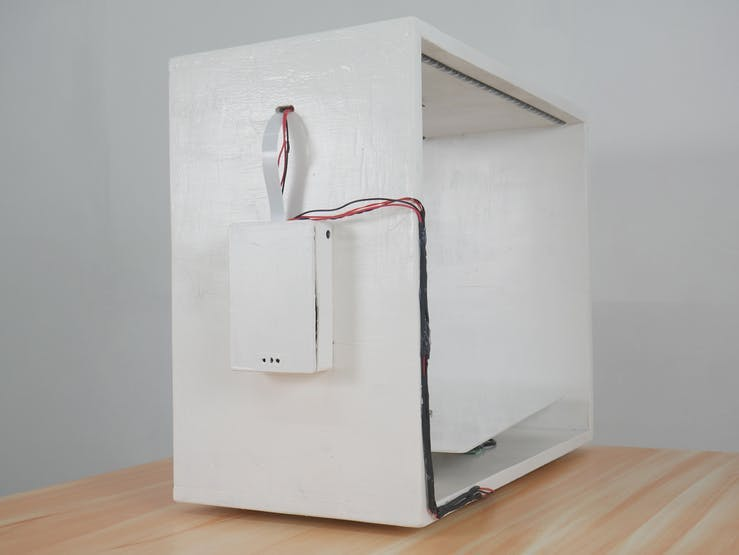### Checkout Interface

The checkout interface has two parts,

1. Front-end developed using HTML, JS

2. Backend API developed using NodeJS and Express

1. Front-end developed using HTML, JS

The front-end continuously checks for the changes happening in the back-end API and displays the changes to the user. Once an item is added to the API, the front-end displays as an item added to the cart.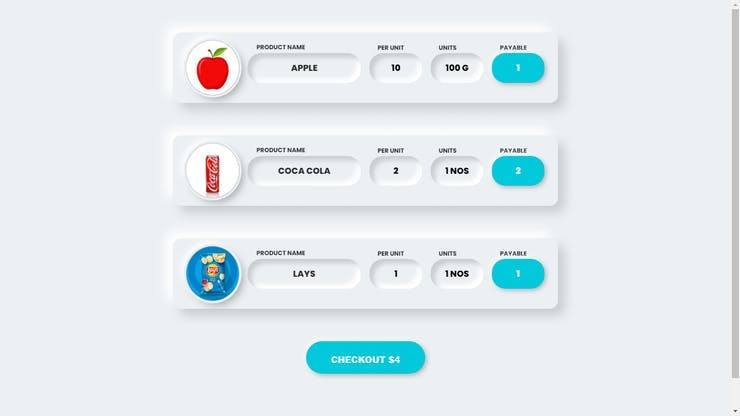2. Backend API developed using NodeJS and Express

The backend REST API is developed using NodeJS and Express. ExpressJS is one of the most popular HTTP server libraries for Node.js, which ships with very basic functionalities. The backend API keeps the details of the products that are visually identified.

For setting our interface we have used a small tablet which is having a touch interface with a small stand.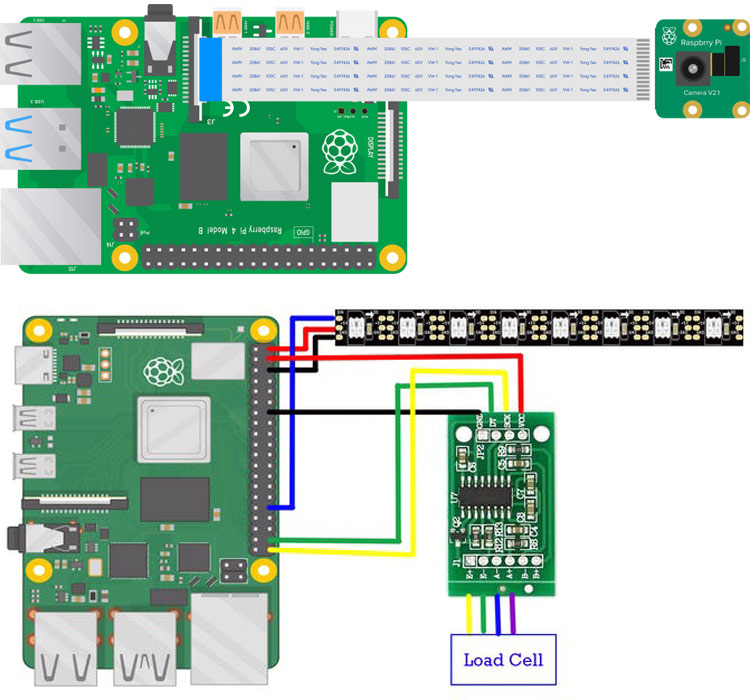##### Code

``` ```

`#!/usr/bin/env python`

``` import cv2 import os import sys, getopt import signal import time from edge_impulse_linux.image import ImageImpulseRunner import RPi.GPIO as GPIO from hx711 import HX711 import requests import json from requests.structures import CaseInsensitiveDict runner = None show_camera = True c_value = 0 flag = 0 ratio = -1363.992 list_label = [] list_weight = [] count = 0 final_weight = 0 taken = 0 a = 'Apple' b = 'Banana' l = 'Lays' c = 'Coke' def now():     return round(time.time() * 1000) def get_webcams():     port_ids = []     for port in range(5):         print("Looking for a camera in port %s:" %port)         camera = cv2.VideoCapture(port)         if camera.isOpened():             ret = camera.read()             if ret:                 backendName =camera.getBackendName()                 w = camera.get(3)                 h = camera.get(4)                 print("Camera %s (%s x %s) found in port %s " %(backendName,h,w, port))                 port_ids.append(port)             camera.release()     return port_ids def sigint_handler(sig, frame):     print('Interrupted')     if (runner):         runner.stop()     sys.exit(0) signal.signal(signal.SIGINT, sigint_handler) def help():     print('python classify.py <path_to_model.eim> <Camera port ID, only required when more than 1 camera is present>') def find_weight():     global c_value     global hx     if c_value == 0:         print('Calibration starts')         try:           GPIO.setmode(GPIO.BCM)           hx = HX711(dout_pin=20, pd_sck_pin=21)           err = hx.zero()           if err:             raise ValueError('Tare is unsuccessful.')           hx.set_scale_ratio(ratio)           c_value = 1         except (KeyboardInterrupt, SystemExit):           print('Bye :)')         print('Calibrate ends')         else :           GPIO.setmode(GPIO.BCM)           time.sleep(1)           try:                 weight = int(hx.get_weight_mean(20))                 #round(weight,1)                 print(weight, 'g')                 return weight           except (KeyboardInterrupt, SystemExit):                 print('Bye :)')                 def post(label,price,final_rate,taken):     global id     id_product = 1     url = "https://automaticbilling.herokuapp.com/product"     headers = CaseInsensitiveDict()     headers["Content-Type"] = "application/json"     data_dict = {"id":id_product,"name":label,"price":price,"unit":"Kg","taken":"1","payable":final_rate}     data = json.dumps(data_dict)     resp = requests.post(url, headers=headers, data=data)     print(resp.status_code)     time.sleep(1)                  def list_com(label,final_weight):     global count     global taken     if final_weight > 2 :            list_weight.append(final_weight)        if count > 1 and list_weight[-1] > list_weight[-2]:            taken = taken + 1     list_label.append(label)     count = count + 1     print('count is',count)     time.sleep(1)     if count > 1:         if list_label[-1] != list_label[-2] :            print("New Item detected")            print("Final weight is",list_weight[-1])            rate(list_weight[-2],list_label[-2],taken)                def rate(final_weight,label,taken):     print("Calculating rate")     if label == a :          print("Calculating rate of",label)          final_rate_a = final_weight * 0.01            price = 10               post(label,price,final_rate_a,taken)     elif label ==b :          print("Calculating rate of",label)          final_rate_b = final_weight * 0.02          price = 20          post(label,price,final_rate_b)     elif label == l:          print("Calculating rate of",label)          final_rate_l = 10          return final_rate     else :          print("Calculating rate of",label)          final_rate_c = final_weight * 0.02          return final_rate def main(argv):     global flag     global final_weight     if flag == 0 :         find_weight()         flag = 1           try:         opts, args = getopt.getopt(argv, "h", ["--help"])     except getopt.GetoptError:         help()         sys.exit(2)     for opt, arg in opts:         if opt in ('-h', '--help'):             help()             sys.exit()     if len(args) == 0:         help()         sys.exit(2)     model = args     dir_path = os.path.dirname(os.path.realpath(__file__))     modelfile = os.path.join(dir_path, model)     print('MODEL: ' + modelfile)     with ImageImpulseRunner(modelfile) as runner:         try:             model_info = runner.init()             print('Loaded runner for "' + model_info['project']['owner'] + ' / ' + model_info['project']['name'] + '"')             labels = model_info['model_parameters']['labels']             if len(args)>= 2:                 videoCaptureDeviceId = int(args)             else:                 port_ids = get_webcams()                 if len(port_ids) == 0:                     raise Exception('Cannot find any webcams')                 if len(args)<= 1 and len(port_ids)> 1:                     raise Exception("Multiple cameras found. Add the camera port ID as a second argument to use to this script")                 videoCaptureDeviceId = int(port_ids)             camera = cv2.VideoCapture(videoCaptureDeviceId)             ret = camera.read()             if ret:                 backendName = camera.getBackendName()                 w = camera.get(3)                 h = camera.get(4)                 print("Camera %s (%s x %s) in port %s selected." %(backendName,h,w, videoCaptureDeviceId))                 camera.release()             else:                 raise Exception("Couldn't initialize selected camera.")             next_frame = 0 # limit to ~10 fps here             for res, img in runner.classifier(videoCaptureDeviceId):                 if (next_frame > now()):                     time.sleep((next_frame - now()) / 1000)                 # print('classification runner response', res)                 if "classification" in res["result"].keys():                     print('Result (%d ms.) ' % (res['timing']['dsp'] + res['timing']['classification']), end='')                     for label in labels:                         score = res['result']['classification'][label]                         if score > 0.9 :                             final_weight = find_weight()                             list_com(label,final_weight)                             if label == a:                                 print('Apple detected')                                    #final_weight = find_weight()                                  elif label == b:                                 print('Banana detected')                             elif label == l:                                 #final_weight = find_weight()                                 print('Lays deteccted')                             else :                                 print('Coke detected')                     print('', flush=True)                 next_frame = now() + 100         finally:             if (runner):                 runner.stop() if __name__ == "__main__":     main(sys.argv[1:]) ..................................................... #!/usr/bin/env python3 import RPi.GPIO as GPIO  # import GPIO from hx711 import HX711  # import the class HX711 try:     GPIO.setmode(GPIO.BCM)  # set GPIO pin mode to BCM numbering     # Create an object hx which represents your real hx711 chip     # Required input parameters are only 'dout_pin' and 'pd_sck_pin'     hx = HX711(dout_pin=20, pd_sck_pin=21)     # measure tare and save the value as offset for current channel     # and gain selected. That means channel A and gain 128     err = hx.zero()     # check if successful     if err:         raise ValueError('Tare is unsuccessful.')     reading = hx.get_raw_data_mean()     if reading:  # always check if you get correct value or only False         # now the value is close to 0         print('Data subtracted by offset but still not converted to units:',               reading)     else:         print('invalid data', reading)     # In order to calculate the conversion ratio to some units, in my case I want grams,     # you must have known weight.     input('Put known weight on the scale and then press Enter')     reading = hx.get_data_mean()     if reading:         print('Mean value from HX711 subtracted by offset:', reading)         known_weight_grams = input(             'Write how many grams it was and press Enter: ')         try:             value = float(known_weight_grams)             print(value, 'grams')         except ValueError:             print('Expected integer or float and I have got:',                   known_weight_grams)         # set scale ratio for particular channel and gain which is         # used to calculate the conversion to units. Required argument is only         # scale ratio. Without arguments 'channel' and 'gain_A' it sets         # the ratio for current channel and gain.         ratio = reading / value  # calculate the ratio for channel A and gain 128         hx.set_scale_ratio(ratio)  # set ratio for current channel         print('Your ratio is', ratio)     else:         raise ValueError('Cannot calculate mean value. Try debug mode. Variable reading:', reading)     # Read data several times and return mean value     # subtracted by offset and converted by scale ratio to     # desired units. In my case in grams.     input('Press Enter to show reading')     print('Current weight on the scale in grams is: ')          print('210.45708403716216 g') except (KeyboardInterrupt, SystemExit):     print('Bye :)') finally:     GPIO.cleanup() .................................................................... """ This file holds HX711 class """ #!/usr/bin/env python3 import statistics as stat import time import RPi.GPIO as GPIO class HX711:     """     HX711 represents chip for reading load cells.     """     def __init__(self,                  dout_pin,                  pd_sck_pin,                  gain_channel_A=128,                  select_channel='A'):         """         Init a new instance of HX711         Args:             dout_pin(int): Raspberry Pi pin number where the Data pin of HX711 is connected.             pd_sck_pin(int): Raspberry Pi pin number where the Clock pin of HX711 is connected.             gain_channel_A(int): Optional, by default value 128. Options (128 || 64)             select_channel(str): Optional, by default 'A'. Options ('A' || 'B')         Raises:             TypeError: if pd_sck_pin or dout_pin are not int type         """         if (isinstance(dout_pin, int)):             if (isinstance(pd_sck_pin, int)):                 self._pd_sck = pd_sck_pin                 self._dout = dout_pin             else:                 raise TypeError('pd_sck_pin must be type int. '                                 'Received pd_sck_pin: {}'.format(pd_sck_pin))         else:             raise TypeError('dout_pin must be type int. '                             'Received dout_pin: {}'.format(dout_pin))         self._gain_channel_A = 0         self._offset_A_128 = 0  # offset for channel A and gain 128         self._offset_A_64 = 0  # offset for channel A and gain 64         self._offset_B = 0  # offset for channel B         self._last_raw_data_A_128 = 0         self._last_raw_data_A_64 = 0         self._last_raw_data_B = 0         self._wanted_channel = ''         self._current_channel = ''         self._scale_ratio_A_128 = 1  # scale ratio for channel A and gain 128         self._scale_ratio_A_64 = 1  # scale ratio for channel A and gain 64         self._scale_ratio_B = 1  # scale ratio for channel B         self._debug_mode = False         self._data_filter = self.outliers_filter  # default it is used outliers_filter         GPIO.setup(self._pd_sck, GPIO.OUT)  # pin _pd_sck is output only         GPIO.setup(self._dout, GPIO.IN)  # pin _dout is input only         self.select_channel(select_channel)         self.set_gain_A(gain_channel_A)     def select_channel(self, channel):         """         select_channel method evaluates if the desired channel         is valid and then sets the _wanted_channel variable.         Args:             channel(str): the channel to select. Options ('A' || 'B')         Raises:             ValueError: if channel is not 'A' or 'B'         """         channel = channel.capitalize()         if (channel == 'A'):             self._wanted_channel = 'A'         elif (channel == 'B'):             self._wanted_channel = 'B'         else:             raise ValueError('Parameter "channel" has to be "A" or "B". '                              'Received: {}'.format(channel))         # after changing channel or gain it has to wait 50 ms to allow adjustment.         # the data before is garbage and cannot be used.         self._read()         time.sleep(0.5)     def set_gain_A(self, gain):         """         set_gain_A method sets gain for channel A.                  Args:             gain(int): Gain for channel A (128 || 64)                  Raises:             ValueError: if gain is different than 128 or 64         """         if gain == 128:             self._gain_channel_A = gain         elif gain == 64:             self._gain_channel_A = gain         else:             raise ValueError('gain has to be 128 or 64. '                              'Received: {}'.format(gain))         # after changing channel or gain it has to wait 50 ms to allow adjustment.         # the data before is garbage and cannot be used.         self._read()         time.sleep(0.5)     def zero(self, readings=30):         """         zero is a method which sets the current data as         an offset for particulart channel. It can be used for         subtracting the weight of the packaging. Also known as tare.         Args:             readings(int): Number of readings for mean. Allowed values 1..99         Raises:             ValueError: if readings are not in range 1..99         Returns: True if error occured.         """         if readings > 0 and readings < 100:             result = self.get_raw_data_mean(readings)             if result != False:                 if (self._current_channel == 'A' and                         self._gain_channel_A == 128):                     self._offset_A_128 = result                     return False                 elif (self._current_channel == 'A' and                       self._gain_channel_A == 64):                     self._offset_A_64 = result                     return False                 elif (self._current_channel == 'B'):                     self._offset_B = result                     return False                 else:                     if self._debug_mode:                         print('Cannot zero() channel and gain mismatch.\n'                               'current channel: {}\n'                               'gain A: {}\n'.format(self._current_channel,                                                     self._gain_channel_A))                     return True             else:                 if self._debug_mode:                     print('From method "zero()".\n'                           'get_raw_data_mean(readings) returned False.\n')                 return True         else:             raise ValueError('Parameter "readings" '                              'can be in range 1 up to 99. '                              'Received: {}'.format(readings))     def set_offset(self, offset, channel='', gain_A=0):         """         set offset method sets desired offset for specific         channel and gain. Optional, by default it sets offset for current         channel and gain.                  Args:             offset(int): specific offset for channel             channel(str): Optional, by default it is the current channel.                 Or use these options ('A' || 'B')                  Raises:             ValueError: if channel is not ('A' || 'B' || '')             TypeError: if offset is not int type         """         channel = channel.capitalize()         if isinstance(offset, int):             if channel == 'A' and gain_A == 128:                 self._offset_A_128 = offset                 return             elif channel == 'A' and gain_A == 64:                 self._offset_A_64 = offset                 return             elif channel == 'B':                 self._offset_B = offset                 return             elif channel == '':                 if self._current_channel == 'A' and self._gain_channel_A == 128:                     self._offset_A_128 = offset                     return                 elif self._current_channel == 'A' and self._gain_channel_A == 64:                     self._offset_A_64 = offset                     return                 else:                     self._offset_B = offset                     return             else:                 raise ValueError('Parameter "channel" has to be "A" or "B". '                                  'Received: {}'.format(channel))         else:             raise TypeError('Parameter "offset" has to be integer. '                             'Received: ' + str(offset) + '\n')     def set_scale_ratio(self, scale_ratio, channel='', gain_A=0):         """         set_scale_ratio method sets the ratio for calculating         weight in desired units. In order to find this ratio for         example to grams or kg. You must have known weight.         Args:             scale_ratio(float): number > 0.0 that is used for                 conversion to weight units             channel(str): Optional, by default it is the current channel.                 Or use these options ('a'|| 'A' || 'b' || 'B')             gain_A(int): Optional, by default it is the current channel.                 Or use these options (128 || 64)         Raises:             ValueError: if channel is not ('A' || 'B' || '')             TypeError: if offset is not int type         """         channel = channel.capitalize()         if isinstance(gain_A, int):             if channel == 'A' and gain_A == 128:                 self._scale_ratio_A_128 = scale_ratio                 return             elif channel == 'A' and gain_A == 64:                 self._scale_ratio_A_64 = scale_ratio                 return             elif channel == 'B':                 self._scale_ratio_B = scale_ratio                 return             elif channel == '':                 if self._current_channel == 'A' and self._gain_channel_A == 128:                     self._scale_ratio_A_128 = scale_ratio                     return                 elif self._current_channel == 'A' and self._gain_channel_A == 64:                     self._scale_ratio_A_64 = scale_ratio                     return                 else:                     self._scale_ratio_B = scale_ratio                     return             else:                 raise ValueError('Parameter "channel" has to be "A" or "B". '                                  'received: {}'.format(channel))         else:             raise TypeError('Parameter "gain_A" has to be integer. '                             'Received: ' + str(gain_A) + '\n')     def set_data_filter(self, data_filter):         """         set_data_filter method sets data filter that is passed as an argument.         Args:             data_filter(data_filter): Data filter that takes list of int numbers and                 returns a list of filtered int numbers.                  Raises:             TypeError: if filter is not a function.         """         if callable(data_filter):             self._data_filter = data_filter         else:             raise TypeError('Parameter "data_filter" must be a function. '                             'Received: {}'.format(data_filter))     def set_debug_mode(self, flag=False):         """         set_debug_mode method is for turning on and off         debug mode.                  Args:             flag(bool): True turns on the debug mode. False turns it off.                  Raises:             ValueError: if fag is not bool type         """         if flag == False:             self._debug_mode = False             print('Debug mode DISABLED')             return         elif flag == True:             self._debug_mode = True             print('Debug mode ENABLED')             return         else:             raise ValueError('Parameter "flag" can be only BOOL value. '                              'Received: {}'.format(flag))     def _save_last_raw_data(self, channel, gain_A, data):         """         _save_last_raw_data saves the last raw data for specific channel and gain.                  Args:             channel(str):             gain_A(int):             data(int):         Returns: False if error occured         """         if channel == 'A' and gain_A == 128:             self._last_raw_data_A_128 = data         elif channel == 'A' and gain_A == 64:             self._last_raw_data_A_64 = data         elif channel == 'B':             self._last_raw_data_B = data         else:             return False     def _ready(self):         """         _ready method check if data is prepared for reading from HX711         Returns: bool True if ready else False when not ready                 """         # if DOUT pin is low data is ready for reading         if GPIO.input(self._dout) == 0:             return True         else:             return False     def _set_channel_gain(self, num):         """         _set_channel_gain is called only from _read method.         It finishes the data transmission for HX711 which sets         the next required gain and channel.         Args:             num(int): how many ones it sends to HX711                 options (1 || 2 || 3)                  Returns: bool True if HX711 is ready for the next reading             False if HX711 is not ready for the next reading         """         for _ in range(num):             start_counter = time.perf_counter()             GPIO.output(self._pd_sck, True)             GPIO.output(self._pd_sck, False)             end_counter = time.perf_counter()             # check if hx 711 did not turn off...             if end_counter - start_counter >= 0.00006:                 # if pd_sck pin is HIGH for 60 us and more than the HX 711 enters power down mode.                 if self._debug_mode:                     print('Not enough fast while setting gain and channel')                     print(                         'Time elapsed: {}'.format(end_counter - start_counter))                 # hx711 has turned off. First few readings are inaccurate.                 # Despite it, this reading was ok and data can be used.                 result = self.get_raw_data_mean(6)  # set for the next reading.                 if result == False:                     return False         return True     def _read(self):         """         _read method reads bits from hx711, converts to INT         and validate the data.                  Returns: (bool || int) if it returns False then it is false reading.             if it returns int then the reading was correct         """         GPIO.output(self._pd_sck, False)  # start by setting the pd_sck to 0         ready_counter = 0         while (not self._ready() and ready_counter <= 40):             time.sleep(0.01)  # sleep for 10 ms because data is not ready             ready_counter += 1             if ready_counter == 50:  # if counter reached max value then return False                 if self._debug_mode:                     print('self._read() not ready after 40 trials\n')                 return False         # read first 24 bits of data         data_in = 0  # 2's complement data from hx 711         for _ in range(24):             start_counter = time.perf_counter()             # request next bit from hx 711             GPIO.output(self._pd_sck, True)             GPIO.output(self._pd_sck, False)             end_counter = time.perf_counter()             if end_counter - start_counter >= 0.00006:  # check if the hx 711 did not turn off...                 # if pd_sck pin is HIGH for 60 us and more than the HX 711 enters power down mode.                 if self._debug_mode:                     print('Not enough fast while reading data')                     print(                         'Time elapsed: {}'.format(end_counter - start_counter))                 return False             # Shift the bits as they come to data_in variable.             # Left shift by one bit then bitwise OR with the new bit.             data_in = (data_in << 1) | GPIO.input(self._dout)         if self._wanted_channel == 'A' and self._gain_channel_A == 128:             if not self._set_channel_gain(1):  # send only one bit which is 1                 return False  # return False because channel was not set properly             else:                 self._current_channel = 'A'  # else set current channel variable                 self._gain_channel_A = 128  # and gain         elif self._wanted_channel == 'A' and self._gain_channel_A == 64:             if not self._set_channel_gain(3):  # send three ones                 return False  # return False because channel was not set properly             else:                 self._current_channel = 'A'  # else set current channel variable                 self._gain_channel_A = 64         else:             if not self._set_channel_gain(2):  # send two ones                 return False  # return False because channel was not set properly             else:                 self._current_channel = 'B'  # else set current channel variable         if self._debug_mode:  # print 2's complement value             print('Binary value as received: {}'.format(bin(data_in)))         #check if data is valid         if (data_in == 0x7fffff                 or  # 0x7fffff is the highest possible value from hx711                 data_in == 0x800000            ):  # 0x800000 is the lowest possible value from hx711             if self._debug_mode:                 print('Invalid data detected: {}\n'.format(data_in))             return False  # rturn false because the data is invalid         # calculate int from 2's complement         signed_data = 0         # 0b1000 0000 0000 0000 0000 0000 check if the sign bit is 1. Negative number.         if (data_in & 0x800000):             signed_data = -(                 (data_in ^ 0xffffff) + 1)  # convert from 2's complement to int         else:  # else do not do anything the value is positive number             signed_data = data_in         if self._debug_mode:             print('Converted 2\'s complement value: {}'.format(signed_data))         return signed_data     def get_raw_data_mean(self, readings=30):         """         get_raw_data_mean returns mean value of readings.         Args:             readings(int): Number of readings for mean. ```

```        Returns: (bool || int) if False then reading is invalid.            ```